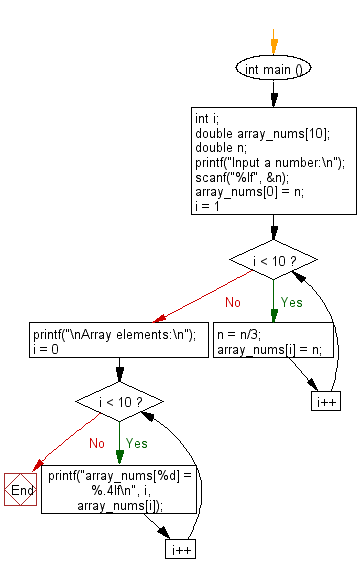﻿ C : One-third of previous value in each position of an array

# C Exercises: Array fill, replace each subsequent position of the array by one-third value of the previous

## C Basic Declarations and Expressions: Exercise-129 with Solution

Write a C program that reads an array (length 10), and replaces the first element of the array by a given number and replaces each subsequent position of the array by one-third the value of the previous.

Sample Solution:

C Code:

``````#include <stdio.h>
int main ()
{
int i;
double array_nums;
double n;

// Prompt user for input
printf("Input a number:\n");
scanf("%lf", &n);

// Initialize the first element of the array with the input value
array_nums = n;

// Loop to generate and store subsequent array elements
for (i = 1; i < 10; i++)
{
n = n / 3;  // Divide the current value by 3
array_nums[i] = n; // Store the result in the array
}

// Print the array elements
printf("\nArray elements:\n");
for (i = 0; i < 10; i++)
{
printf("array_nums[%d] = %.4lf\n", i, array_nums[i]);
}

return 0; // End of program
}
``````

Sample Output:

```Input a number:
35

Array elements:
array_nums = 35.0000
array_nums = 11.6667
array_nums = 3.8889
array_nums = 1.2963
array_nums = 0.4321
array_nums = 0.1440
array_nums = 0.0480
array_nums = 0.0160
array_nums = 0.0053
array_nums = 0.0018
```

Flowchart:C programming Code Editor:

What is the difficulty level of this exercise?

Test your Programming skills with w3resource's quiz.

﻿